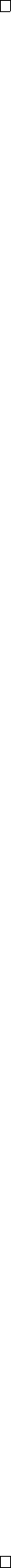3Integration

II Probability and Measure3.2 Integrals and limits
We are now going to prove more things about exchanging limits and integrals.
These are going to be extremely useful in the future, as we want to exchange
limits and integrals a lot.
Theorem
(Fatou’s lemma)
.
Let (
f
n
) be a sequence of non-negative measurable
functions. Then
µ(lim inf f
n
) lim inf µ(f
n
).
Note that a special case was proven in the first example sheet, where we did
it for the case where f
n
are indicator functions.
Proof.
k n
, then we always have
that
inf
mn
f
m
f
k
.
By the monotonicity of the integral, we know that
µ
inf
mn
f
m
µ(f
k
).
for all k n.
So we have
µ
inf
mn
f
m
inf
kn
µ(f
k
) lim inf
m
µ(f
m
).
It remains to show that the left hand side converges to
µ
(
lim inf f
m
). Indeed,
we know that
inf
mn
f
m
% lim inf
m
f
m
.
Then by monotone convergence, we have
µ
inf
mn
f
m
% µ
lim inf
m
f
m
.
So we have
µ
lim inf
m
f
m
lim inf
m
µ(f
m
).
No one ever remembers which direction Fatou’s lemma goes, and this leads to
many incorrect proofs and results, so it is helpful to keep the following example
in mind:
Example. We let (E, E, µ) = (R, B, Lebesgue). We let
f
n
= 1
[n,n+1]
.
Then we have
lim inf
n
f
n
= 0.
So we have
µ(f
n
) = 1 for all n.
So we have
lim inf µ(f
n
) = 1, µ(lim inf f
n
) = 0.
So we have
µ
lim inf
m
f
m
lim inf
m
µ(f
m
).
The next result we want to prove is the dominated convergence theorem.
This is like the monotone convergence theorem, but we are going to remove the
increasing and non-negative measurable condition, and add in something else.
Theorem
(Dominated convergence theorem)
.
Let (
f
n
)
, f
be measurable with
f
n
(
x
)
f
(
x
) for all
x E
. Suppose that there is an integrable function
g
such
that
|f
n
| g
for all n, then we have
µ(f
n
) µ(f)
as n .
Proof. Note that
|f| = lim
n
|f|
n
g.
So we know that
µ(|f|) µ(g) < .
So we know that f, f
n
are integrable.
Now note also that
0 g + f
n
, 0 g f
n
for all
n
. We are now going to apply Fatou’s lemma twice with these series. We
have that
µ(g) + µ(f) = µ(g + f)
= µ
lim inf
n
(g + f
n
)
lim inf
n
µ(g + f
n
)
= lim inf
n
(µ(g) + µ(f
n
))
= µ(g) + lim inf
n
µ(f
n
).
Since µ(g) is finite, we know that
µ(f) lim inf
n
µ(f
n
).
We now do the same thing with g f
n
. We have
µ(g) µ(f) = µ(g f)
= µ
lim inf
n
(g f
n
)
lim inf
n
µ(g f
n
)
= lim inf
n
(µ(g) µ(f
n
))
= µ(g) lim sup
n
µ(f
n
).
Again, since µ(g) is finite, we know that
µ(f) lim sup
n
µ(f
n
).
These combine to tell us that
µ(f) lim inf
n
µ(f
n
) lim sup
n
µ(f
n
) µ(f).
So they must be all equal, and thus µ(f
n
) µ(f).